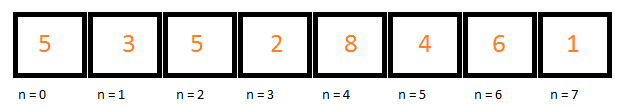# Part 3.4Arrays in YASS

ZPE has two structures that are very similar but differ in the way they work. Firstly, the list type.

## ZPE lists

A list in ZPE (the offical underlying type is called the ZPEList) is a structure that stores multiple sub-variables inside it. A list can be of any size. A list assigns an index to each value, so the first index is the 0th index, then 1st index, 2nd index and so on. Each value inside a list or an array is called an element.ZPE lists start at index 0 (n=0). In this example 8 numbers have been stored in this list.

The following code sample demonstrates how to declare an empty list:

YASS
$arr = []  This list is empty. It is also easy to declare an list full of values: YASS $arr = ["Jamie", "Bonnar", "Balfour"]


Accessing items is also done with the square brackets:

YASS
$arr = ["Jamie", "Bonnar", "Balfour"] print($arr)


This will print the value Balfour as it is the value found in the element with index 2.

Items can also be appended to a list using a similar syntax. The following sample demonstrates this:

YASS
$arr = ["Jamie", "Bonnar", "Balfour"]$arr = "James"
$arr = "Bond" print($arr)
print($arr)  But the position for which the index is for that element does not need to be the next one in the array: YASS $arr = ["Jamie", "Bonnar", "Balfour"]
$arr = "James"$arr = "Bond"
print($arr)  The result of this is several null values are added: [Jamie, Bonnar, Balfour, null, null, James, Bond] A null value (represented in ZPE/YASS as null) means that a memory location has been left empty. In otherwords, a null value has no actual methods, properties or functions that work with it associated with it. ## ZPE arrays ZPE's array type was added in ZPE 1.6.7 in October 2018. Since then it has not changed too much and it actually based upon the ZPEList data type. The official underlying data type is called ZPEArray. ZPE arrays are ultimately fixed length lists. When they are created, they are given a size: YASS $arr = [] * 5
print($arr)  Ultimately this will allocate 5 memory slots but will fill them with null values: [null, null, null, null, null] To get round this and make it it is possible to set the initial value of each element. For example an array of numbers each value should initially be set to the number 0. The following sample demonstrates this: YASS $arr =  * 5
print($arr)  Likewise, with an array of string each element should initially be set to an empty string: YASS $arr = [""] * 5
print($arr)  The size of an array cannot be changed the way that a list can be and accessing an element that is outwith the size of the array will cause a runtime error: YASS $arr = [""] * 5
print(\$arr)


This will result in error being displayed and the program exiting:

Runtime exception occured in 'main' => "Index out of range: Index: 6, Size: 5"

Further, the type initially set into the array cannot be changed. So if the array was declared as above with five empty strings and a number was inserted, the ZPE runtime would crash.

Feedback 👍
Comments are sent via email to me.